# 978-0123869449 Chapter 14

Document Type
Homework Help
Book Title
Authors
Michael F. Modest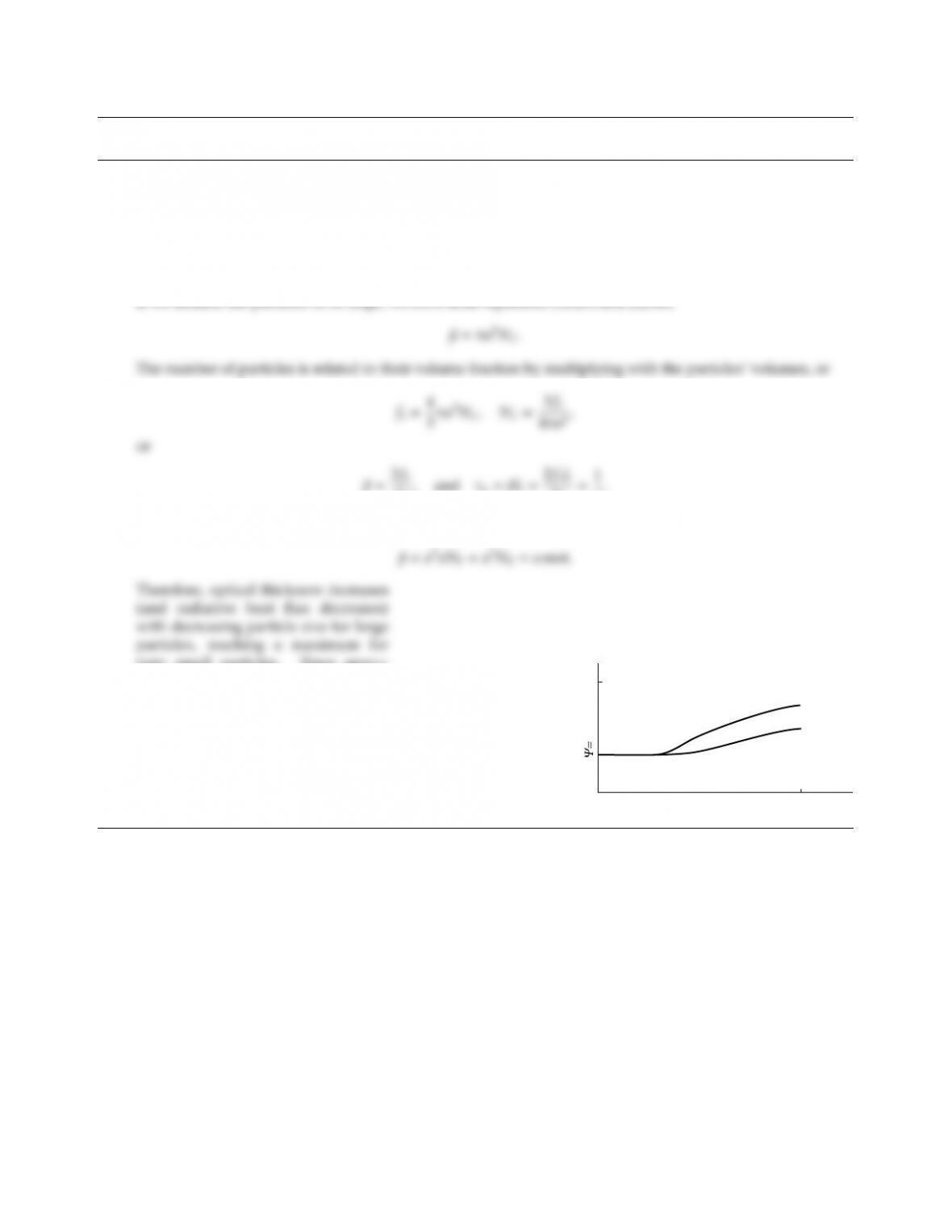CHAPTER 14
14.1 The gap between two parallel black plates at T1and T2, respectively, is filled with a particle-laden gas.
from two dierent materials (one a specular reﬂector, the other a diuse reﬂector, both having the same ǫ).
Sketch the nondimensional heat ﬂux Ψ = q(T4
1T4
2) vs. particle size (but keeping volume-fraction constant).
Solution
If we assume the particles to be large, we have from equations (12.29) and (12.66)
4a,and τL=βL=2fvL
4a1
a.
For small particles Rayleigh scattering applies, and from equations (12.29) and (12.46), or from equation (12.53),
very small particles. Since specu-
lar spheres have a forward scattering
peak (see Fig. 12-13) more energy is
transmitted from plate to plate than for
diuse spheres, which have a strong
backward scattering peak. Since acan-
not increase beyond a single large par-
ticle, the heat ﬂux cannot increase be-
yond a certain point.
0
q/
σ
(T
1
4
T
4
)
2
amax
diffuse
specular
a
360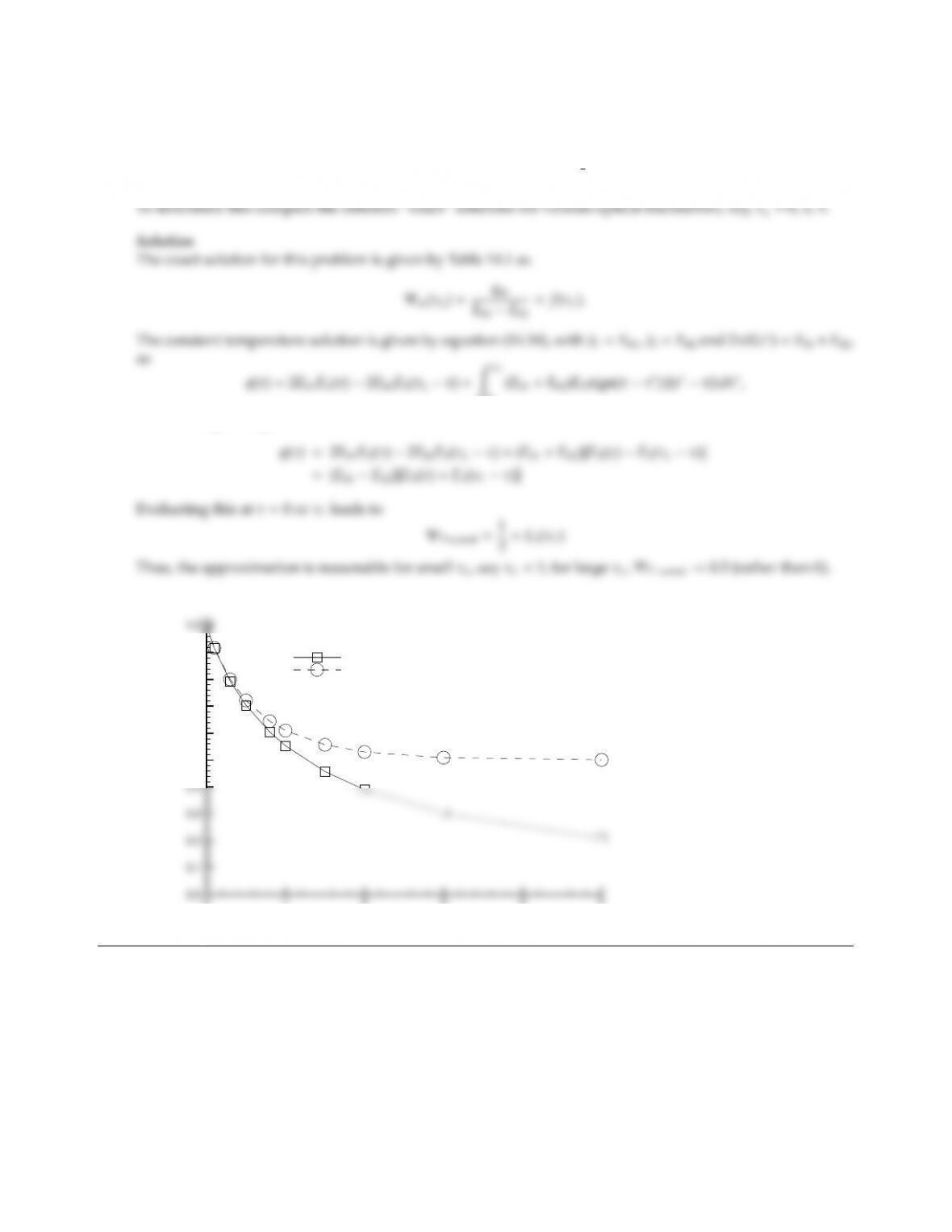CHAPTER 14 361
14.2 Consider radiative equilibrium in a one-dimensional, gray, nonscattering, plane-parallel medium bounded
by isothermal black plates at temperatures T1and T2. To make a simple closed-form solution possible to
determine the heat ﬂux between the plates, it has been proposed to replace the radiative-equilibrium slab by a
constant temperature slab, with its temperature evaluated at T4
av =1
2(T4
1+T4
2).Under what optical conditions
is this a good idea, if ever?
0
or, following Example 14.1:
0 1 2 3 4 5
τL
0.5
0.6
0.7
0.8
0.9
Ψ
Constant temperature slab at Tav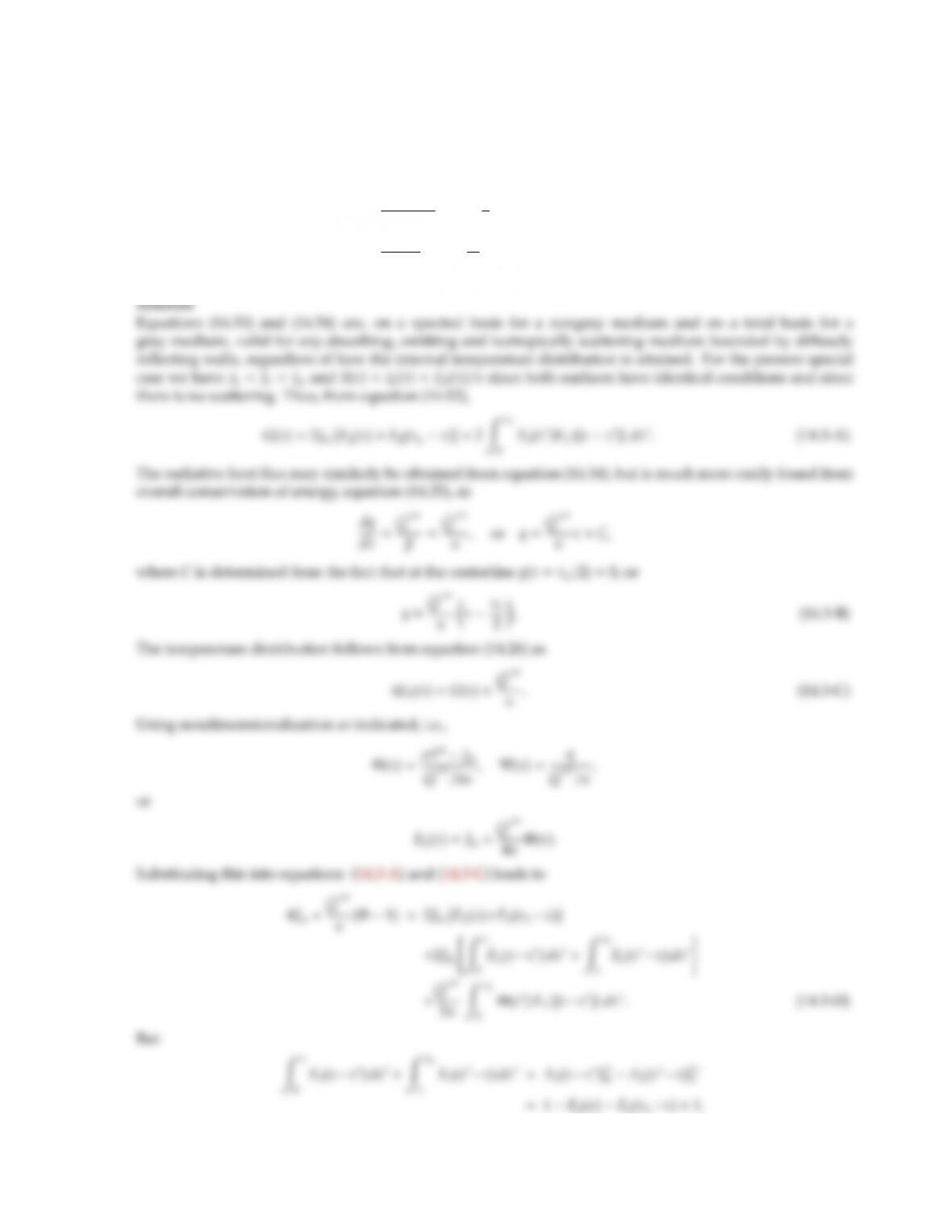14.3 Consider a space enclosed by infinite, diuse-gray parallel plates filled with a gray nonscattering medium.
The surfaces are isothermal (both at Tw), and there is uniform and constant heat generation within the
medium per unit volume, ˙
Q′′′. Conduction and convection are negligible so that ·q=˙
Q′′′. Set up the
integral equations describing temperature and heat ﬂux distribution in the enclosure, i.e., show that
Φ(τ)=σT4Jw
˙
Q′′′/4κ=1+1
2ZτL
0
Φ(τ)E1(|ττ|)dτ,
Ψ(τ)=q
˙
Q′′′=ττL
2.2.
Once Φhas been determined the radiosity is related to wall temperature through equation (14.48), or
Q′′′
τL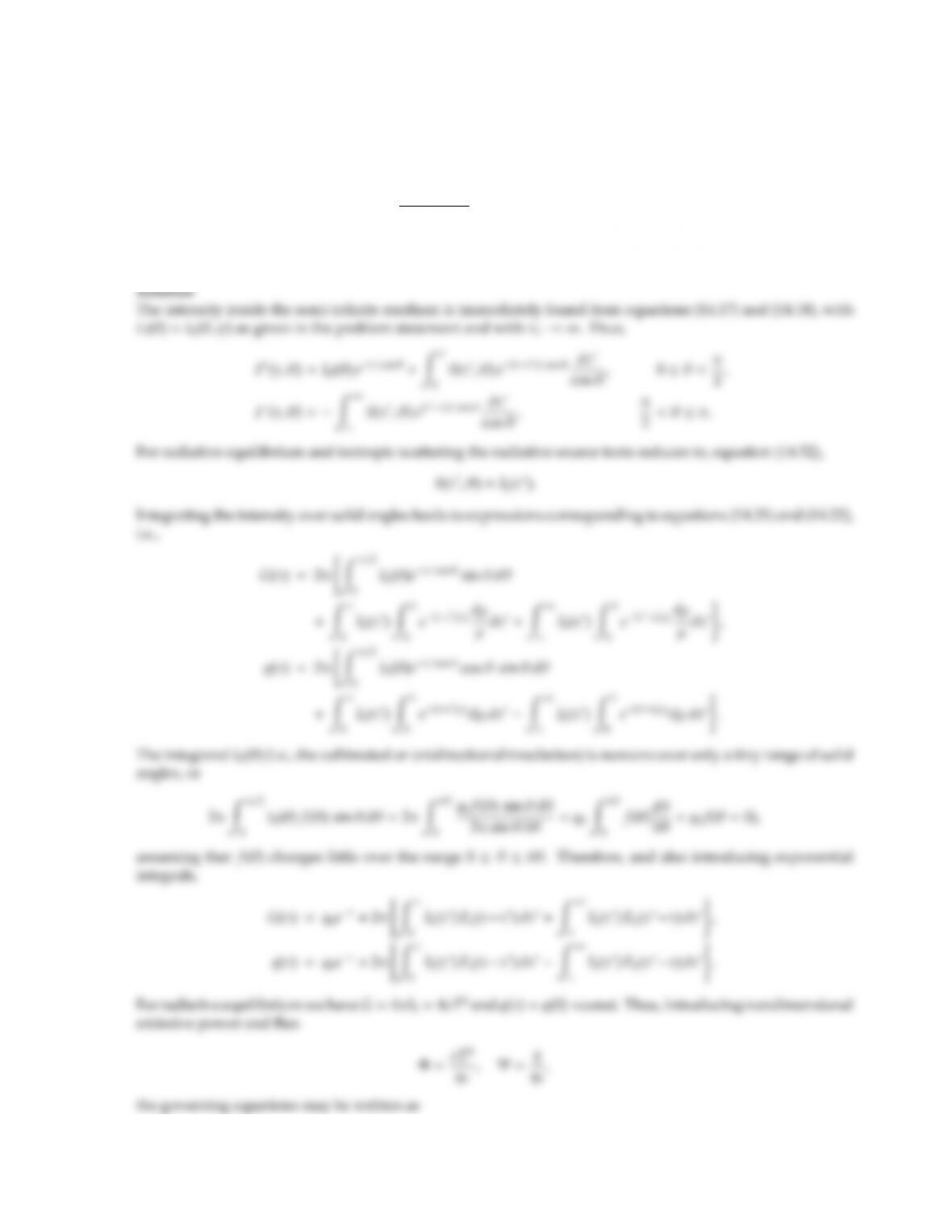14.4 A semi-infinite, gray, isotropically scattering medium, originally at a temperature of 0 K, is subjected to
collimated irradiation with a constant heat ﬂux q0normal to its nonreﬂecting surface. Set up the integral
relationships governing steady-state temperature and radiative heat ﬂux within the medium, assuming
I0(θ, ψ)=
q0
2πsin θ δθ,0θ < δθ, 0ψ2π,
0,elsewhere.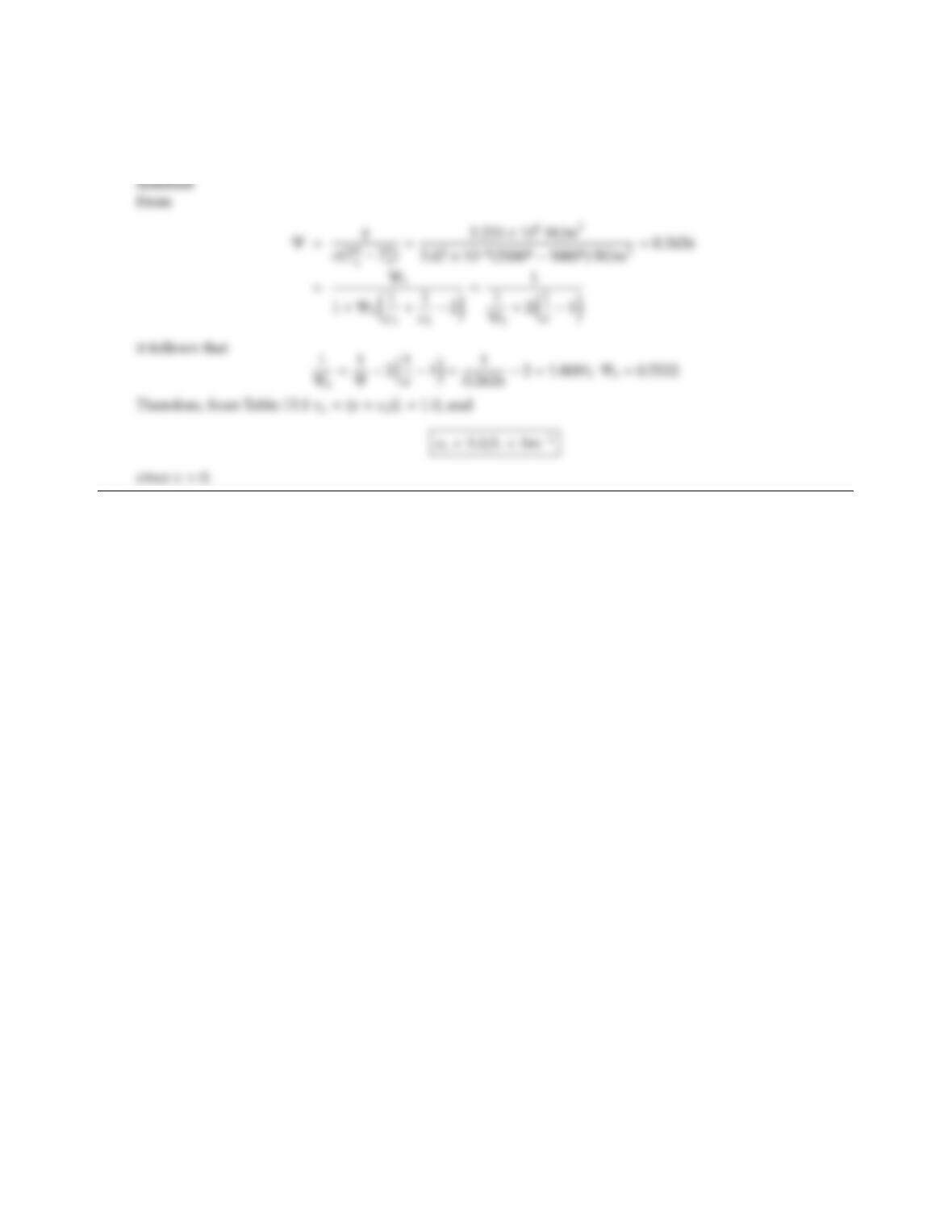14.5 Two large, isothermal gray plates at T1=2000 K, T2=1000 K with ǫ=ǫ1=ǫ2=0.5 are separated by a gap
of width L=1 m filled with purely (isotropically) scattering particles. If the heat ﬂux between the plates has
been measured as 223.3 kW/m2, what is the medium’s scattering coecient?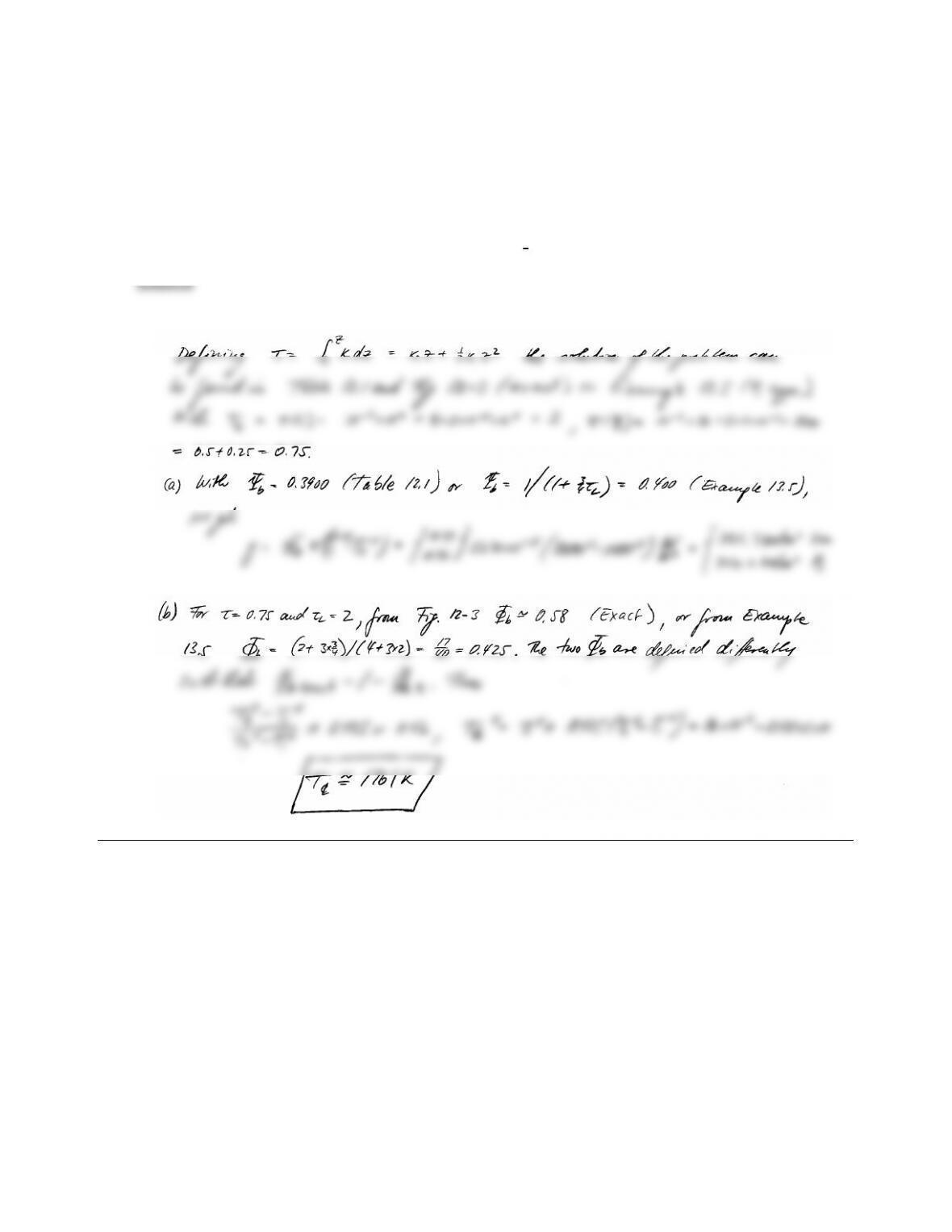CHAPTER 14 367
14.6 Consider two parallel, black, isothermal plates spaced 1 m apart with T1=2000 K and T2=1000 K. The
medium between the plates is gray and at radiative equilibrium with a nonconstant absorption coecient of
κ=κ0+κ
1z;κ0=102cm1, κ
1=2×104cm2.
The medium does not scatter.
(a) What is the heat ﬂux between the plates?
(b) What is the temperature at the medium’s center (z=1
2L)?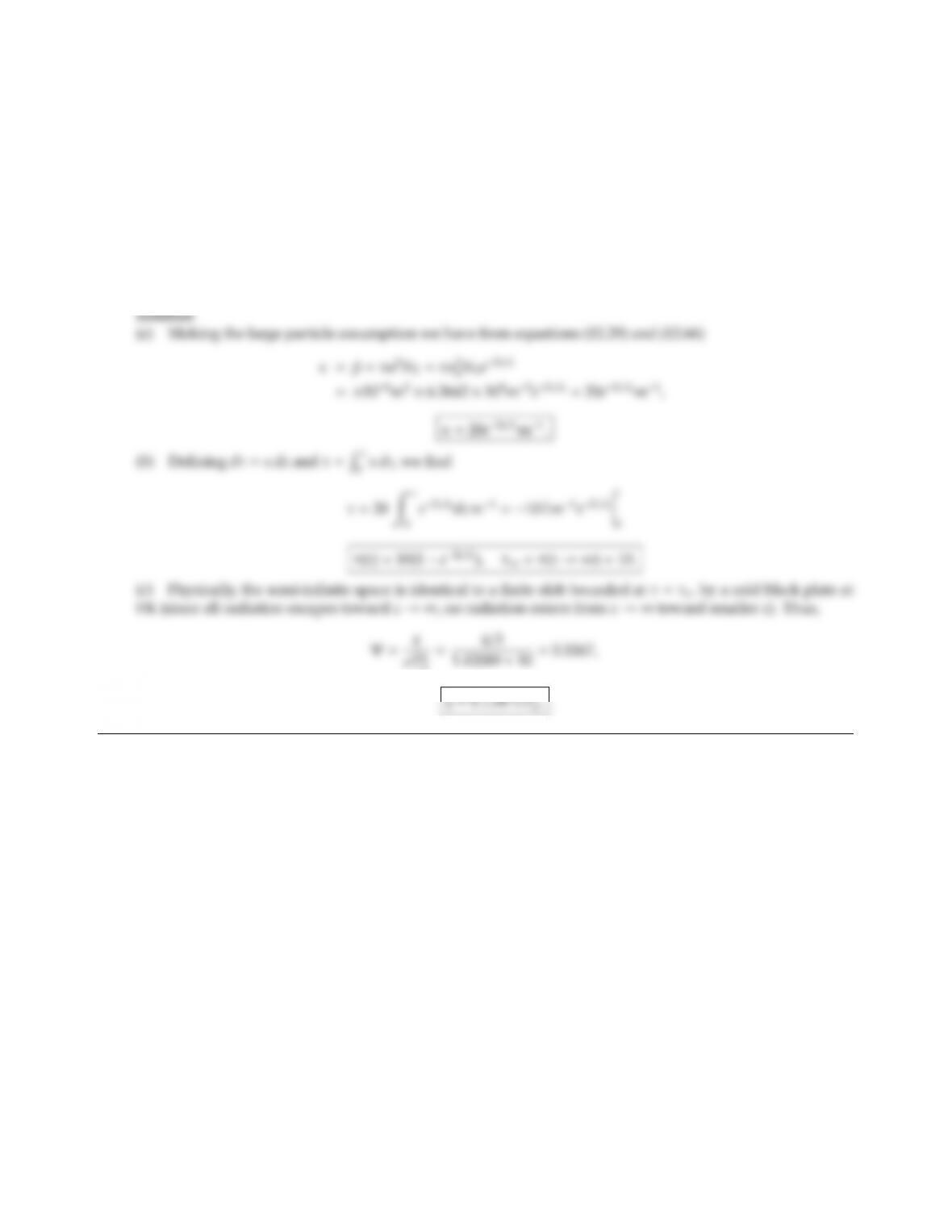14.7 An infinite, black, isothermal plate bounds a semi-infinite space filled with black spheres. At any given
distance, z, away from the plate the particle number density is identical, namely NT=6.3662 ×108m3.
However, the radius of the suspended spheres diminishes monotonically away from the surface as
a=a0ez/L;a0=104m,L=1 m.
(a) Determine the absorption coecient as a function of z(you may make the large-particle assumption).
(b) Determine the optical coordinate as a function of z. What is the total optical thickness of the semi-infinite
space?
(c) Assuming that radiative equilibrium prevails, determine the heat loss from the plate.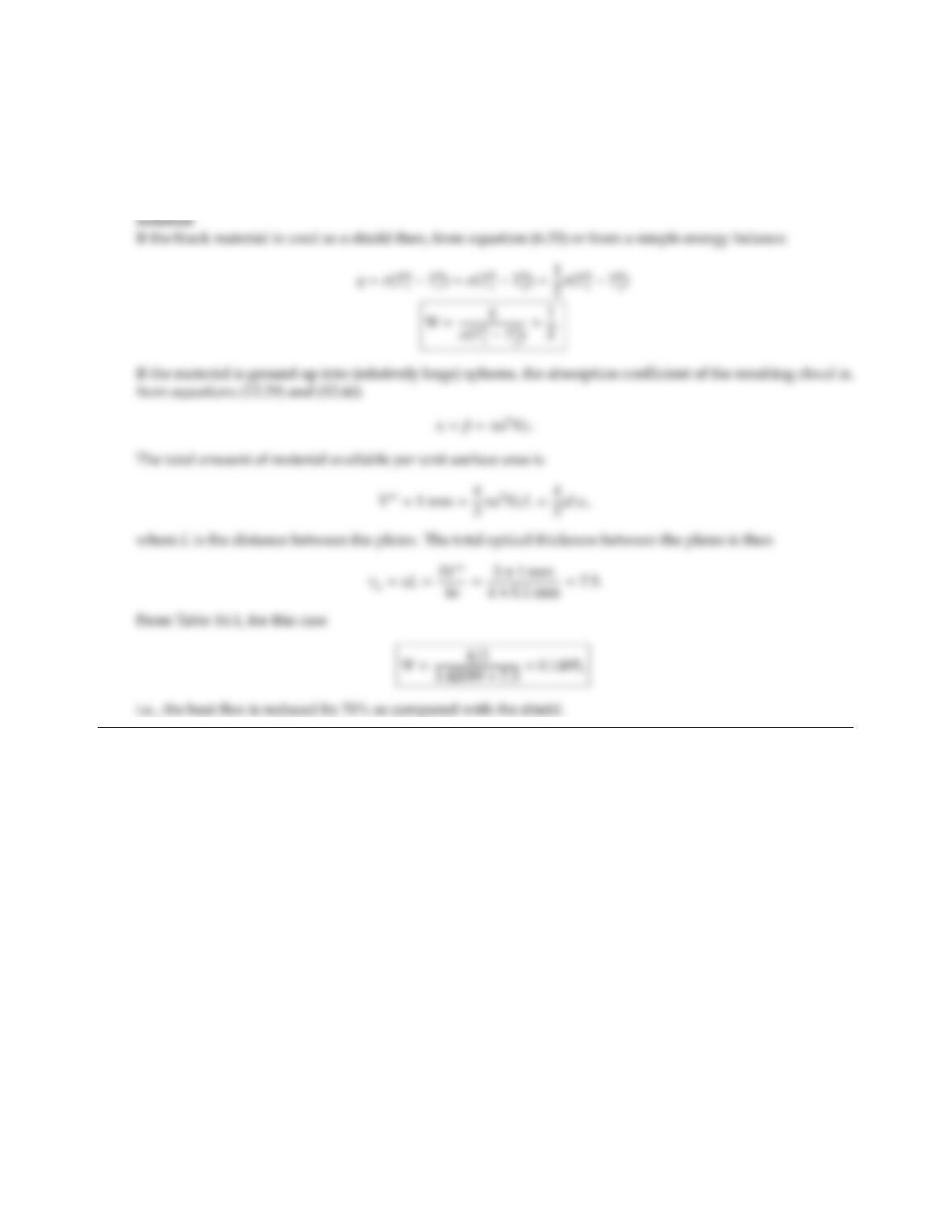CHAPTER 14 369
14.8 The radiative heat transfer between two isothermal, black plates at temperatures T1and T2and separated
by a nonparticipating gas is to be minimized. Enough of a black material is available to place a 1 mm thick
radiation shield between the plates. Alternatively, the same amount of material could be used in the form of
small spheres of 0.1 mm radius to be suspended between the plates. Which possibility results in lower heat
ﬂux, assuming conduction and convection to be negligible?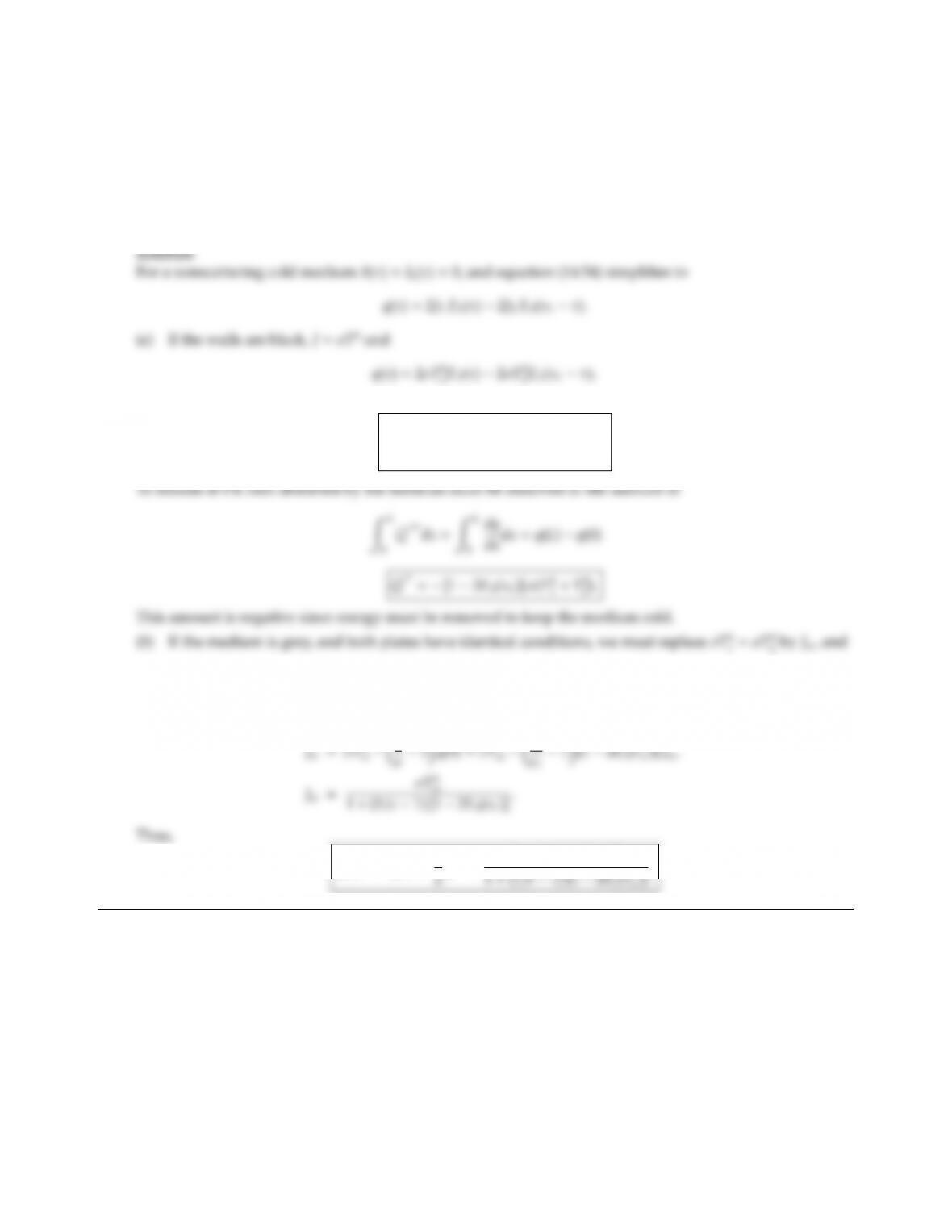14.9 Two infinite, isothermal plates at temperatures T1and T2are separated by a cold, gray medium of optical
thickness τL=κL(no scattering).
(a) Calculate the radiative heat ﬂux at the bottom plate, the top plate, and the net radiative energy going
into the gray medium, assuming that both plates are black.
(b) Repeat (a), but assume that both plates have the same temperature T, and that both plates are gray with
equal emittance ǫ(diuse emission and reﬂection).
or
q(0) =σT4
12σT4
2E3(τL),
q(L)=hσT4
22σT4
1E3(τL)i.
q(0) =q(L)=Jw[12E3(τL)].
The radiosity is determined from equation (14.48) as
q(0) =q(L)=1
Q′′ =[12E3(τL)]σT4
w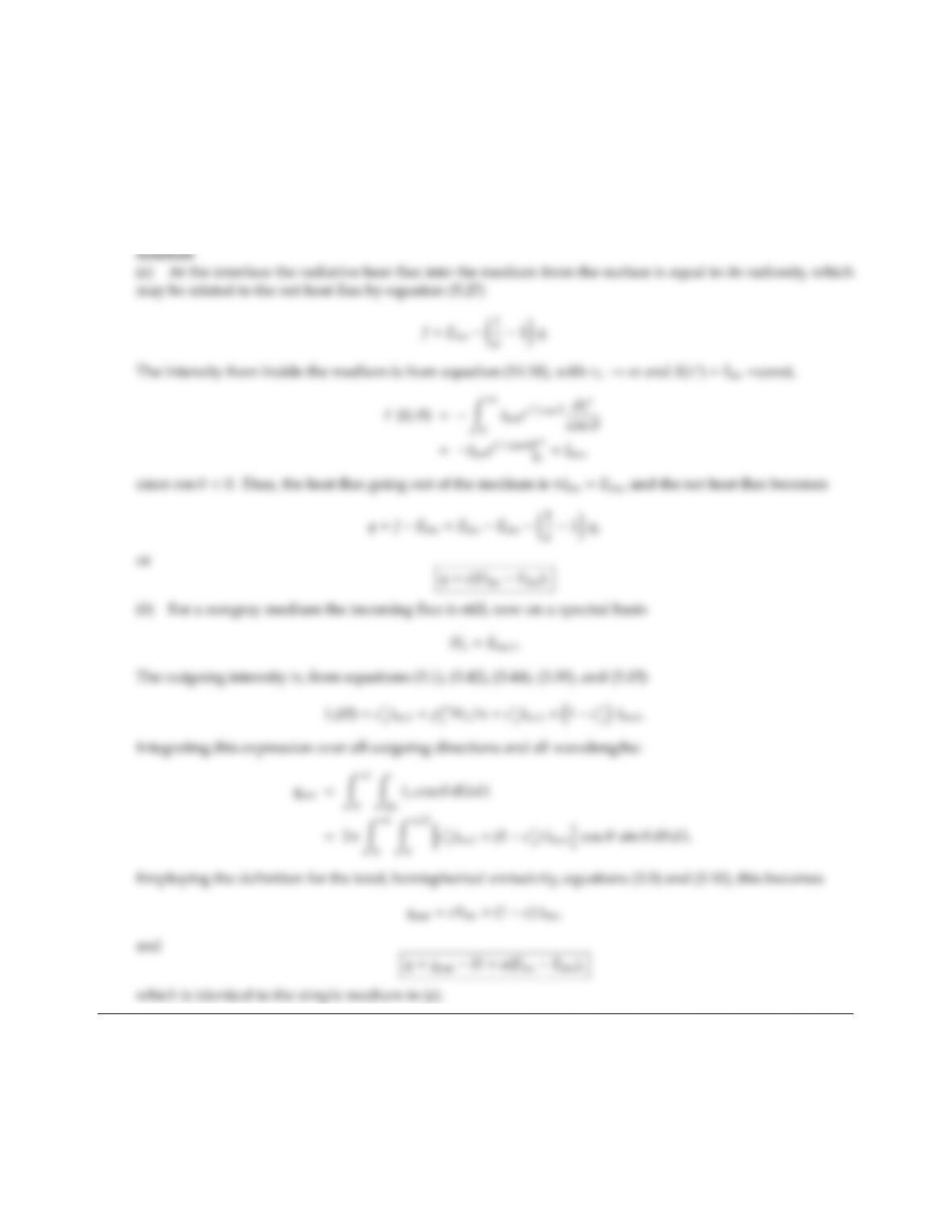CHAPTER 14 371
14.10 A semi-infinite, absorbing-emitting, nonscattering medium at uniform temperature is in contact with a gray-
diuse wall at Twand with emittance ǫw.
(a) The medium is gray, and has a constant absorption coecient. Determine the net radiative heat ﬂux at
the wall.
(b) Let the medium be nongray with nonconstant absorption coecient κλ, and the wall be nongray and
nondiuse with spectral, directional emittance ǫ
λ. How would this aect the wall heat ﬂux?14.11 A 1 m thick, isothermal slab bounded by two cold black plates has a temperature of 3000 K, and a nongray
absorption coecient that can be approximated by
κλ=(0, λ < 2µm,
0.20 cm1, λ > 2µm.
Calculate the total heat loss by radiation from the slab (in W/cm2).
=0.20×100
The total heat loss from top and bottom is
2|q(0)|=2×5.670 ×108×30004W
m2[1 0.73778][1 2×0] =2.41 ×106W/m2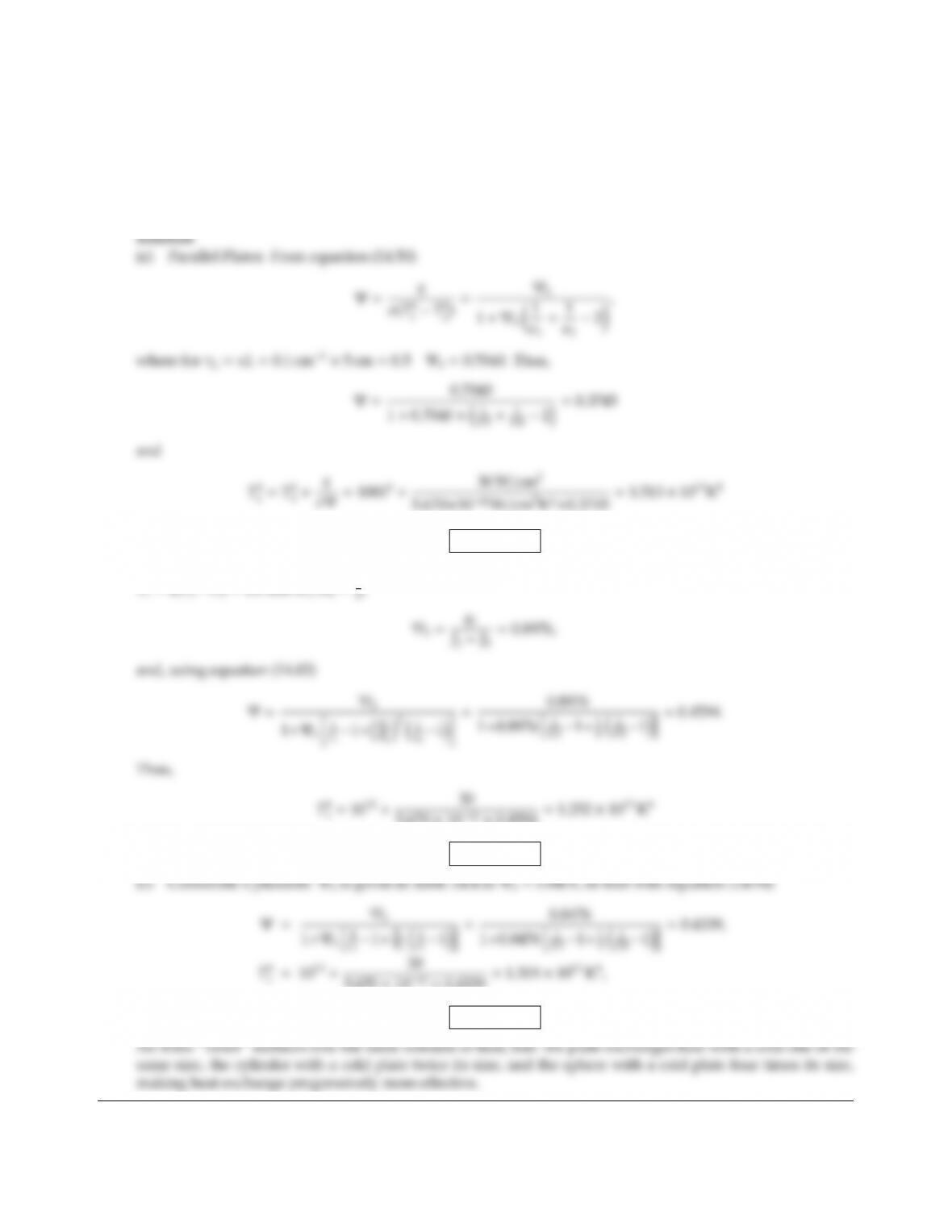CHAPTER 14 373
14.12 Consider (a) two parallel plates, (b) two concentric spheres, (c) two concentric cylinders. The bottom/inner
surface needs to dissipate a heat ﬂux of 30 W/cm2and has a gray-diuse emittance ǫ1=0.5. The top/outer
surface is at T2=1000 K with ǫ2=0.8. The medium in between the surfaces is gray and nonscattering
(κ=0.1 cm1), has a thickness of L=5 cm and is at radiative equilibrium. Determine the temperature at the
bottom/inner surface necessary to dissipate the supplied heat for the three dierent cases (the radii of the
inner cylinder and sphere are R1=5 cm). Discuss the results.
T1=1972 K.
(b) Concentric Spheres: In the absence of heat generation we have, from equation (14.75) and Table 14.2 for
τL=2(τ2τ1)=1.0 and R1/R2=1
5.670 ×1012 ×0.4594 =1.252 ×1013 K4
T1=1881 K.
5.670 ×1012 ×0.4339 =1.319 ×1013 K4,
T1=1906 K.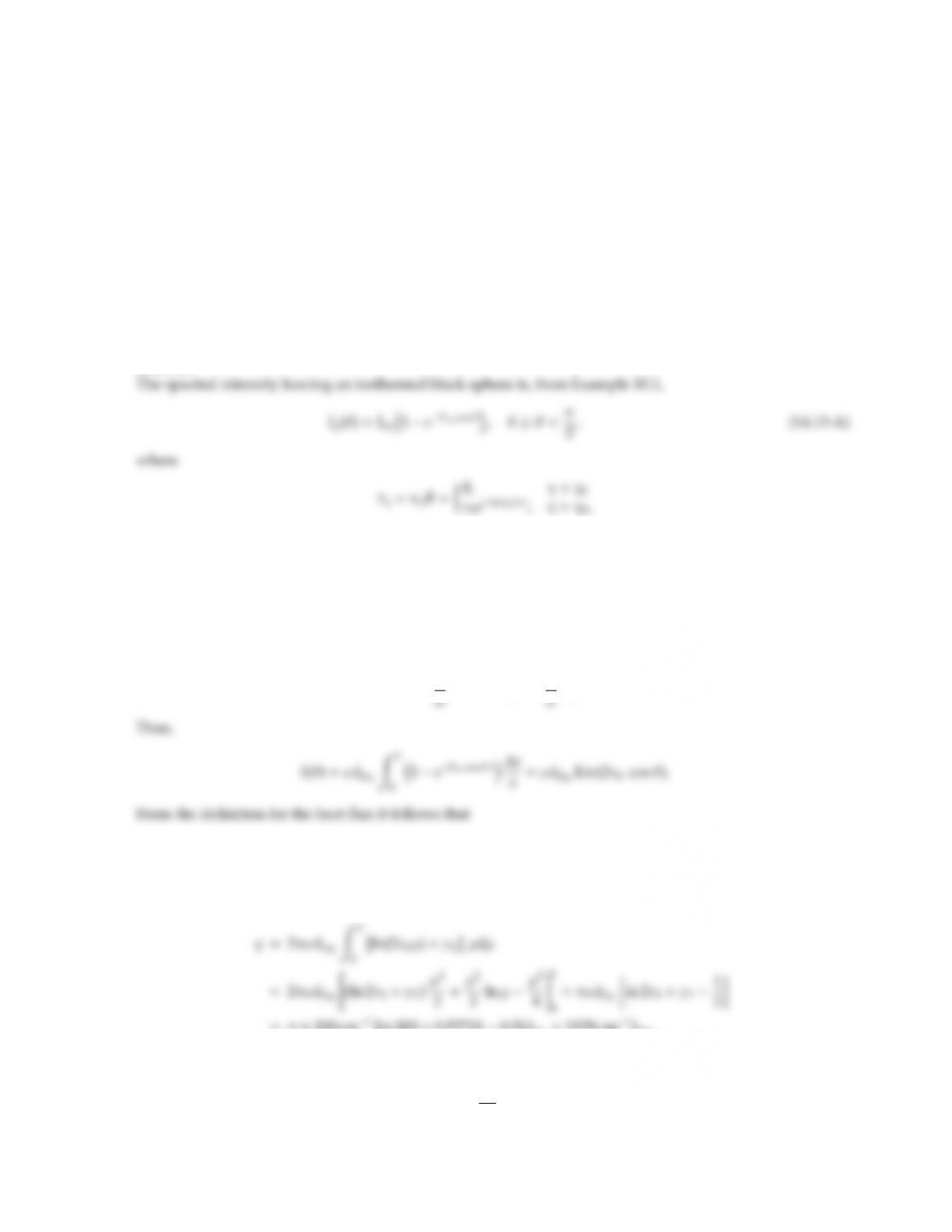14.13 Consider a very hot sphere of a nongray gas of radius R=1 m in 0 K surroundings that have been evacuated.
The gas has a single absorption-emission band in the infrared, with an absorption coecient
κη=(0, η < 3000 cm1=η0,
κ0e(ηη0), η > 3000 cm1,
where κ0=1 cm1, ω =200 cm1. During cool-down the sphere is always isothermal, and remains of constant
size (i.e., constant density ρ=1000 g/m3). The heat capacity of the gas is cp=1 kJ/kg K. Determine the time
required to cool the gas from Ti=6000 K to Te=1000 K. Sketch qualitatively the behavior of Ψ = qT4vs. T.
Hint: To make an analytical solution possible, you may make the following assumptions:
(a) Ein(x)=R1
(b) Wien’s distribution may be used.
Solution
and τ0=κ0R=1 cm1×1 m =100. Integrating equation (14.13-A) over the spectrum (assuming the band to
be narrow enough to extract Ibηfrom the integral) leads to
I(θ)=Z
0
Iηdη=Ibη0Z
η01e2τηcos θdη.
Changing the integration variable to x=τη0we obtain
dx =1
q=2πZπ/2
0
I(θ) cos θsin θdθ=2πZ1
0
ωIbη0Ein(2τ0µ)µdµ.
Since τ0µ1 wherever µis appreciable, we may replace this by
=π×200 cm1[ln 200 +0.57721 0.5]Ibη0=3378 cm1Ibη0.
From a heat balance, assuming radiation to be the sole mechanism for the sphere to lose heat:
ρcpVdT
dt =Aq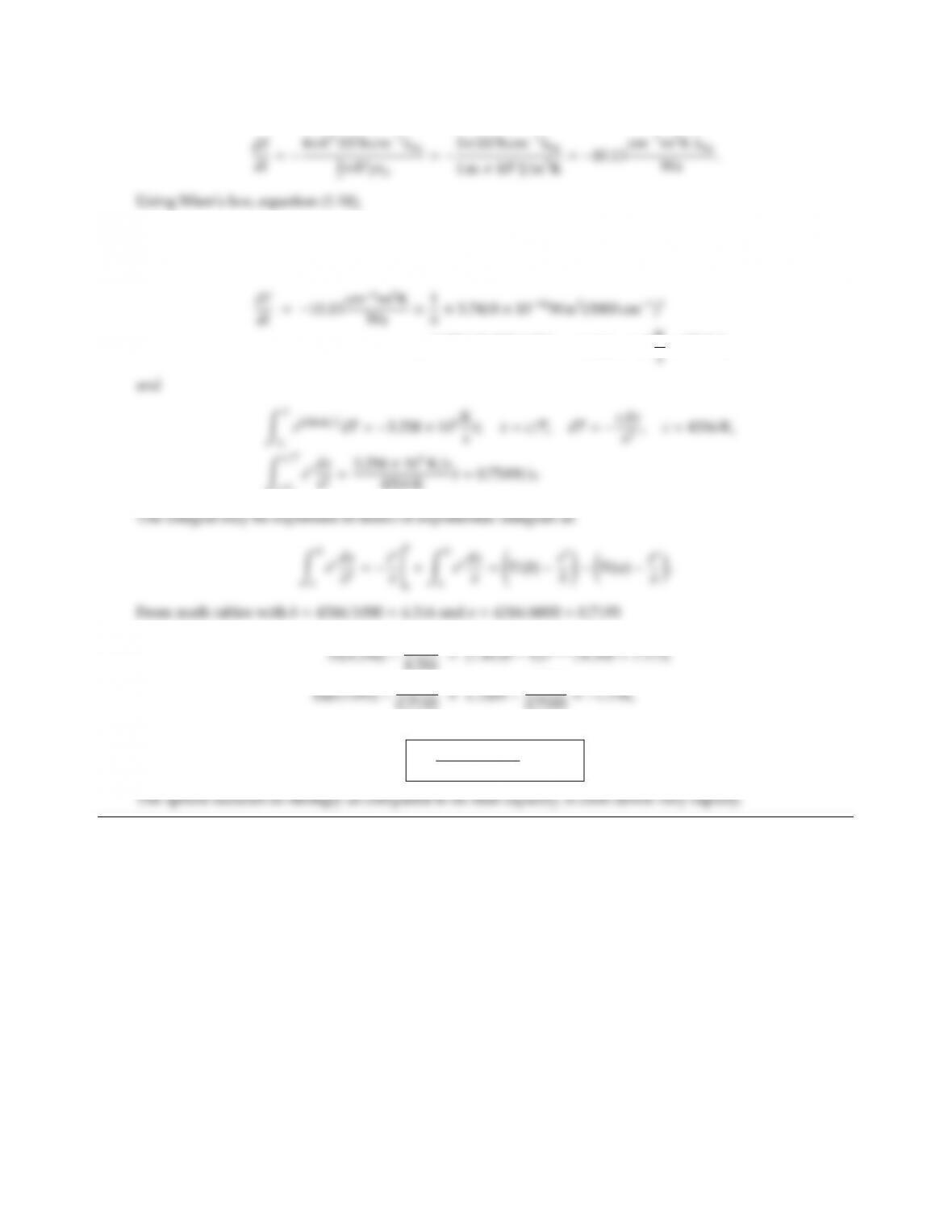CHAPTER 14 375
or
Ebη0=Ebλ02
0=C1η3
0eC2η0/T,
so that
×e1.4388 cmK×3000 cm1/T=3.258 ×103K
c/Ti
4.316 =(1.4135 1) e4.316/4.316 =7.175,
0.7193 =1.1203 e0.7193
0.7193 =1.734,
and
t=7.175 +1.734
0.7549 =11.8 s.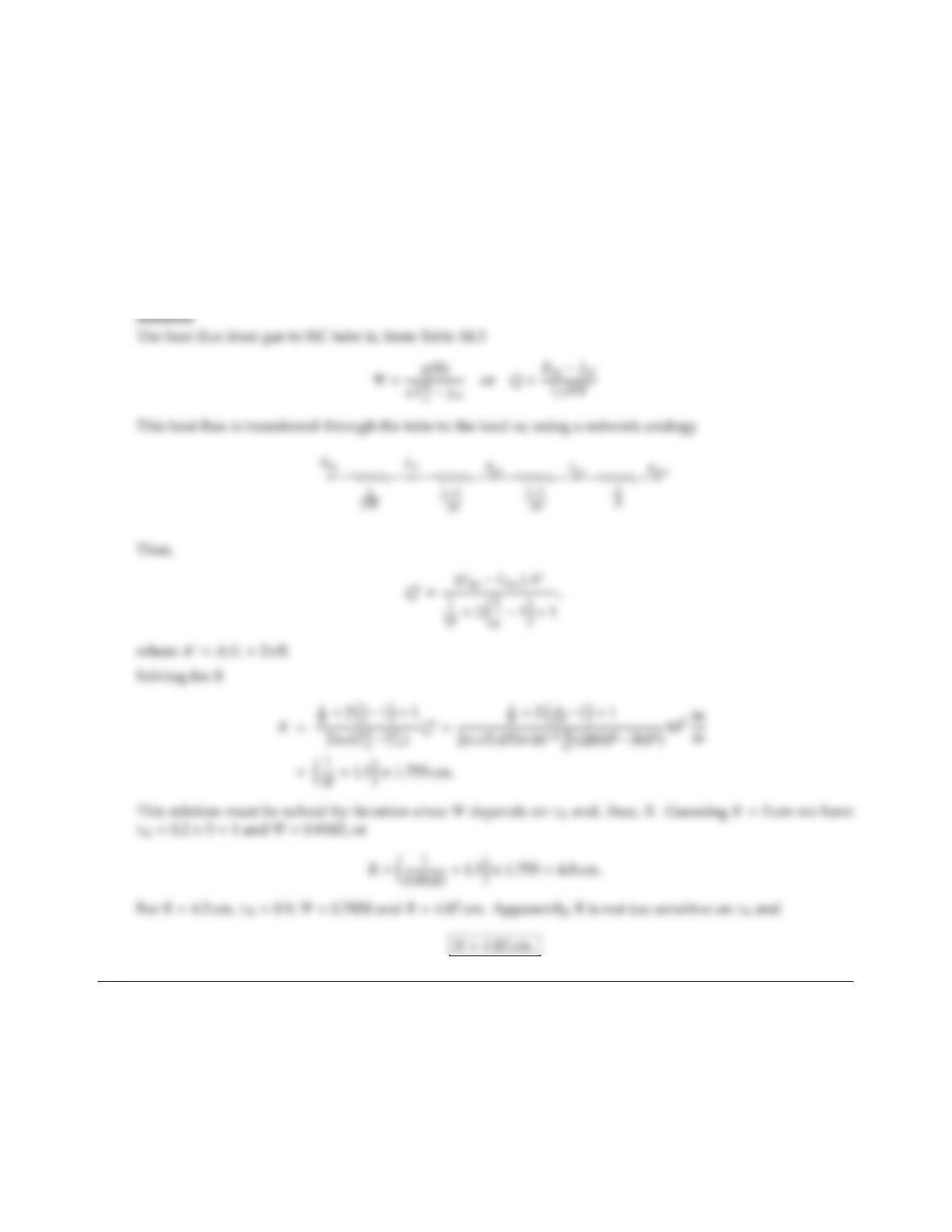14.14 It is proposed to construct a high-temperature heating element by guiding hot combustion gases through a
silicon carbide tube. The outside of the SiC tube then radiates heat toward the load. Such devices are known
as “radiant tubes.” For the design of such a radiant tube you may make the following assumptions:
(i) the combustion gas inside the radiant tube is essentially gray and isothermal with κ=0.2 cm1and
Tgas =2000 K;
(ii) the silicon carbide tube wall is essentially isothermal and of negligible thickness, with a gray-diuse
emittance of 0.8 on both sides,
(iii) the long tube is contained in a large furnace with a background temperature of 1000 K.
Determine the necessary tube diameter to achieve a radiant heating rate of 100 kW per m length of tube.

## Trusted by Thousands ofStudents

Here are what students say about us.